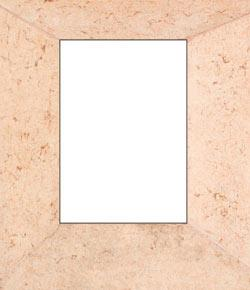# Square Numbers

10 Questions | Total Attempts: 872SettingsMaths questions mainly about Square Numbers. There may be some square root or division problems as well.

• 1.
Solve the equation described. Nine squared minus seven squared, divide the answer by eight, then find the square root of that answer.
• A.

2

• B.

4

• C.

32

• D.

49

• E.

81

• 2.
What does twelve squared equal?
• A.

24

• B.

36

• C.

144

• D.

44

• E.

14

• 3.
What does thirteen squared equal?
• A.

156

• B.

13 squares

• C.

158

• D.

169

• E.

131

• 4.
What does fifteen squared equal?
• A.

250

• B.

15 squares

• C.

235

• D.

215

• E.

225

• 5.
What does seventeen squared equal?
• A.

290

• B.

291

• C.

293

• D.

297

• E.

289

• 6.
What does eighteen squared equal?
• A.

324

• B.

1818

• C.

321

• D.

318

• E.

325

• 7.
What does 31 squared equal?
• A.

931

• B.

952

• C.

961

• D.

991

• E.

992

• 8.
What does 45 squared equal?
• A.

2045

• B.

4545

• C.

2090

• D.

2025

• E.

2035

• 9.
What does 56 squared equal?
• A.

3156

• B.

3166

• C.

3126

• D.

3146

• E.

3136

• 10.
What does 114 squared equal?
• A.

12,996

• B.

14,144

• C.

12,997

• D.

12,998

• E.

12,999

Related Topics